# Texas Go Math Grade 4 Lesson 15.2 Answer Key Customary Units of Length

Refer to our Texas Go Math Grade 4 Answer Key Pdf to score good marks in the exams. Test yourself by practicing the problems from Texas Go Math Grade 4 Lesson 15.2 Answer Key Customary Units of Length.

## Texas Go Math Grade 4 Lesson 15.2 Answer Key Customary Units of Length

Essential Question

How can you convert customary units of length?
The customary system of measurement is defined as a set of Weights and measures used for measuring length, weight, capacity, and temperature. Based on the English system of measurement, it is also known as the U.S. Customary System

Unlock the problem

You can use a ruler to measure length. A ruler that is 1 foot long shows 12 inches in 1 foot. A ruler that is 3 feet long is called a yardstick. There are 3 feet in 1 yard.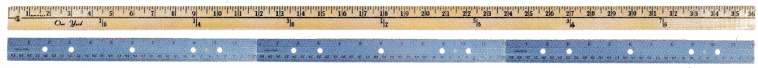How does the size of a foot compare to the size of an inch?Activity

Materials

• 1-inch grid paper
• scissors
• tape

STEP 1 Cut out the paper-inch tiles. Label each tile 1 inch.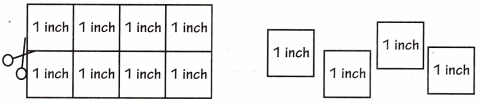STEP 2 Place 12 tiles end-to-end to build 1 foot. Tape the tiles together.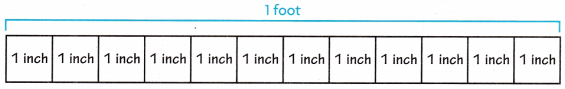STEP 3 Compare the size of 1 foot to the size of 1 inch.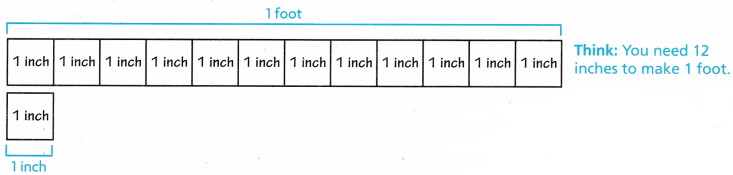So, 1 foot is ____________ times as long as 1 inch.
So, 1 foot is 12 times as long as 1 inch.
Explanation:
1 foot = 12 inches
3 feet = 1 yard

Math Talk

Mathematical Processes
How many inches would you need to make a yard?
32 inches make a yard
Explanation:
3 feet = 1 yard
1 foot = 12 inches
so,
3 x 12 = 36inches.

Example 1 Convert larger units to smaller units.

Emma has 4 feet of thread to make some bracelets. How many inches of thread does Emma have?

Since 1 foot is 12 times as long as 1 inch, you can write feet as inches by multiplying the number of feet by 12.

Make a table that relates feet and inches.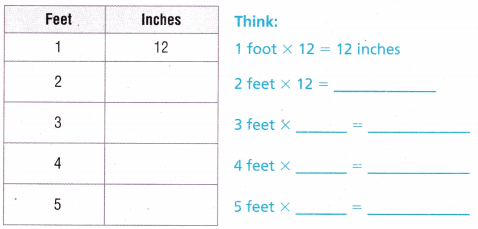So, Emma has ___________ inches of thread.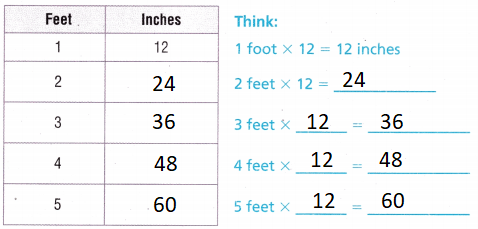Explanation:
Each feet is multiplied with 12
for converting into inches
So, Emma has 48 inches of thread.

Example 2 Convert smaller units to larger units.

Jason ran 300 feet during recess. How many yards did Jason run?

Make a table that relates feet and yards.So, Jason ran ____________ yards during recess.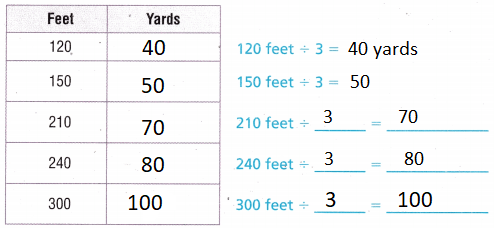Explanation:
3 feet = 1 yard
300 ÷3 = 100
So, Jason ran 100 yards during recess.

What if Emma had 5 feet of thread? Would she have enough thread to make the bracelets? Explain.
yes, she has
Explanation:
5 feet = 60 inches.
she has to made bracelet with 4 feet only
so, there will be 1 feet remaining.

Math Talk

Mathematical Processes
Explain how making a table helped you solve the problem.
The conversions are directly noted in the table
There will be no need of calculating each time
Explanation:
With the help of table we have to just analyze and answer.

Share and Show

Question 1.
Compare the size of a yard to the size of a foot.
Use a model to help.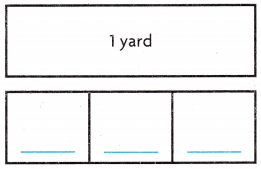1 yard is _________ times as long as _________ foot.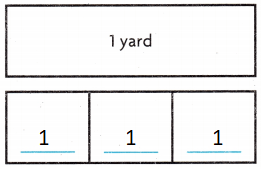Explanation:
1 yard is 3 times as long as 1 foot.

Complete.

Question 2.
2 feet = _________ inches
2 feet = 24 inches
Explanation:
1 foot = 12 inches
2 x 12 = 24 inches

Question 3.
3 yards = _________ feet
3 yards = 9 feet
Explanation:
1 yard = 3 feet
3 x 3 = 9

Question 4.
12 feet = _________ yards
12 feet = 4 yards
Explanation:
3 feet = 1 yard
12 ÷  3 = 4

Question 5.
7 yards = _________ feet
7 yards = 21 feet
Explanation:
1 yard = 3 feet
7 x  3 = 21 feet

Question 6.
144 inches = _________ feet
144 inches = 12 feet
Explanation:
1 feet = 12 inches
144 ÷ 12 = 12 feet

Question 7.
72 feet = _________ yards
72 feet = 24 yards
Explanation:
3 feet = 1 yard
72 ÷ 3 = 24 yards

Math Talk

Mathematical Processes
If you measured the length of your classroom in yards and then in feet, which unit would have a greater number of units? Explain.
Yards
Explanation:
Measuring by yards are better than in feet
because 1 yard = 3 feet.

Problem Solving

Question 8.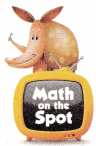H.O.T. Multi-Step Joanna has 3 yards of fabric. She needs 100 inches of fabric to make curtains. Does she have enough fabric to make curtains? Explain. Make a table to help.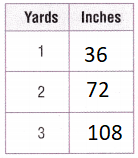Explanation:
1 yard = 3 feet
3 x 3 = 9 feet
1 feet = 12 inches
12 x 9 = 108 inches
With the help of table we can easily know that
Joanna has enough fabric.

Question 9.
H.O.T. Justify Hannah’s room is 14 feet wide. She says that her room is 160 inches wide. Is Hannah correct? Explain your answer.
168 > 160 so, Hannah correct
Explanation:
1 feet = 12 inches
14 = 168 inches
14 x 12 = 168 inches.

Question 10.
A spool of thread contains 6 yards of thread. How many feet of thread are on the spool?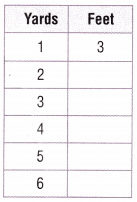(A) 6 feet
(B) 9 feet
(C) 18 feet
(D) 2 feet
6 yards = 18 feet
Explanation:Question 11.
Which statement about 8 yards and 25 feet is true?
(A) 25 feet = 8 yards
(B) 25 feet < 8 yards
(C) 8 yards > 25 feet
(D) 8 yards < 25 feet
Explanation:
1 yard = 3 feet
8 x 3 =24 feet

Question 12.
Multi-Step Carla has 3 feet of ribbon. She needs 38 inches of ribbon to wrap a present. How many more inches of ribbon does Carla need?
(A) 2 inches
(B) 35 inches
(C) 29 inches
(D) 3 inches
Explanation:
3 feet = 36 inches
38 – 36 = 2 inches
2 more inches of ribbon that Carla need

TEXAS Test Prep

Question 13.
Jim is putting carpet in his basement. The length of his basement is 12 yards. How many feet is the length of his basement?
(A) 4 feet
(B) 15 feet
(C) 36 feet
(D) 432 feet
Explanation:
12 yards
1 yard = 3 feet
12 x 3 = 36 feet
36 feet is the length of his basement

### Texas Go Math Grade 4 Lesson 15.2 Homework and Practice Answer Key

Complete.Question 1.
3 feet = ___________ inches
3 feet = 36 inches
Explanation:
1 feet = 12 inches
12 x 3 = 36

Question 2.
5 yards = ___________ inches
5 yards = 180 inches
Explanation:
1 yard = 3 feet
1 feet = 12 inches
5 x 3 = 15 feet
15 x 12 = 180 inches

Question 3.
4 yards = ___________ feet
4 yards = 12 feet
Explanation:
1 yard = 3 feet
4 x 3 = 12 feet

Question 4.
72 inches = ___________ yards
72 inches = 2 yards
Explanation:
1 yard = 3 feet
1 feet = 12 inches
72 ÷ 12 = 6
6 ÷ 3 = 2

Question 5.
9 feet = ___________ yards
9 feet = 3 yards
Explanation:
1 yard = 3 feet
1 feet = 12 inches
9 ÷ 3 = 3

Question 6.
60 inches = ___________ feet
60 inches = 5 feet
Explanation:
1 yard = 3 feet
1 feet = 12 inches
60 ÷ 12 = 5

Problem Solving

Question 7.
Conor has a roll of fence that is 25 feet long. He needs 8 yards of fence to go around a flower bed. Does he have enough fence to go around the flower bed? Explain. Make a table to help you.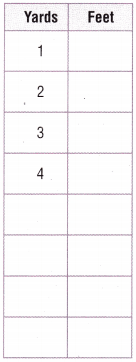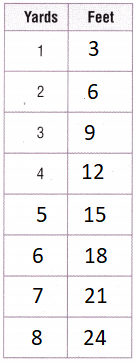Explanation:
Conor has a roll of fence that is 25 feet long.
He needs 8 yards of fence to go around a flower bed.
He does not have enough fence to go around the flower bed
8 yard = 24 feet.

Question 8.
If you measured the width of a window in inches and then in feet, which measurement would have the greater number of units? Explain your answer.
Feet
Explanation:
Measuring by feet are better than in inches
because 1 feet = 12 inches.

Question 9.
Ginny’s house is 20 yards long. She says that the house is 720 feet long. Is Ginny correct? Explain your answer.
1 yard = 3 feet
Explanation:
20 yards = 60 feet
so, Ginny is not correct

Lesson Check

Question 10.
Which statement is true?
(A) 15 feet = 3 yards
(B) 15 yards = 3 feet
(C) 15 feet = 5 yards
(D) 15 yards = 5 feet
Explanation:
15 feet = 5 yards
is the correct statement.

Question 11.
The statement Evan wrote comparing 60 inches and 6 feet is true. Which statement did he write?
(A) 60 inches = 6 feet
(B) 60 inches > 6 feet
(C) 6 feet < 60 inches
(D) 6 feet > 60 inches
Explanation:
6 feet = 72 inches
6 feet < 60 inches
This is the correct statement.

Question 12.
Halley has a 5-foot long extension cord. She wants to know its length in inches. How many inches long is the cord?
(A) 60 inches
(B) 180 inches
(C) 15 inches
(D) 48 inches
Explanation:
1 feet = 36 inches
5 x 36 = 180 inches
180 inches long is the cord

Question 13.
Alex’s driveway is 27 yards long and 10 yards wide. How many feet long is the driveway?
(A) 9 feet
(B) 81 feet
(C) 972 feet
(D) 324 feet
Explanation:
27 yard = 81 feet
1 yard = 3 feet
27 x 3 = 81 feet

Question 14.
Multi-Step A roll of tape is 50 yards long. How many pieces of tape 2 inches long can be cut from the tape?
(A) 150
(B) 9,000
(C) 900
(D) 300
Explanation:
1 yard = 36 inches
50 x 36 = 1800 inches
1800 ÷ 2 = 900 inches

Question 15.
Multi-Step Vicky has a 50 inch roll of ribbon. She uses 3 feet of the ribbon to wrap a gift. How many inches of ribbon does she have left?
(A) 4 inches
(B) 41 inches
(C) 14 inches
(D) 24 inches